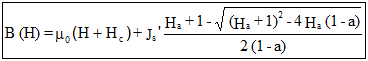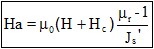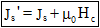# Magnet (unidirectional): demagnetization curve (Hc, Br) + knee

## Presentation

This model ( Nonlinear magnet described by H c and the B r module, plus knee adjustment ) defines a nonlinear B(H) dependence with taking into account of demagnetization and knee adjustment .

Main characteristics:

• the mathematical model and the direction of magnetization are dissociated
• a single material for description of several regions

## Mathematical model

In the direction of magnetization the model is a combination of a straight line and an arc tangent curve . A coefficient adjusts the shape of the curve knee for a better approximation of the experimental curve

The corresponding mathematical formula is written:withwhere:

• μ0 is the permeability of vacuum, μ0 = 4 π 10-7 H/m
• μr is the relative permeability of material (for H = Hc)
• Hc is the coercive field strength A/m
• a is the knee adjustment coefficient (0 < a and a < > 1)

The smaller the coefficient, the sharper the knee is.

• Js' is computed by dichotomy from Br, Hc and μr, with the equationThe shape of the B(H) dependence in the direction of magnetization is given in the opposite figure.In transversal directions one can write:

B(H) = μ0μr⊥H

where μr⊥ is the transverse relative permeability

## Direction of magnetization

The various possibilities provided to the user are the same ones as those presented in § Magnet (unidirectional): linear approximation.